< Challenges of energy rating of bifacial and BIPV modules

# Summary of Standards Procedures for Bifacial Parameters Measurements and Calculations

George Koutsourakis, James Blakesley; National Physical Laboratory (NPL), United Kingdom

# 1. Introduction

The procedures for the measurement of the current-voltage (I-V) characteristics and bifaciality parameters of bifacial photovoltaic devices are analytically described in the IEC 60904-1-2 document . A short summary of these procedures and a bifacial parameter calculation example are provided below.

# 2. Bifaciality coefficients

Bifaciality is quantified with reference to bifaciality coefficients: the short-circuit current bifaciality coefficient φIsc, the open-circuit voltage bifaciality coefficient φVoc and the maximum power bifaciality coefficient φPmax.

As described in IEC 60904-1-2, for single-sided illumination measurements of bifacial PV devices, a solar simulator (as defined in IEC 60904-9 ) with adjustable irradiance level has to be used for the I-V characterisation. Irradiance levels above 1000 Wm-2 (typically up to 1200 Wm-2) need to be achieved, non-uniformity of irradiance must be below 5 % for all irradiance levels used for the characterisation of bifacial devices. For double-sided illumination a solar simulator with the capability to simultaneously illuminate the bifacial device on both sides must be used. Irradiance at different levels on both sides needs to be provided with the non-uniformity of irradiance being below 5 % on both sides.

In order to accurately measure the I-V characteristics of each side of bifacial PV devices, the amount of light incident on the opposite side of the device under test must be eliminated completely during the measurement by creating a non-irradiated background. The background is considered to be non-irradiating if the irradiance incident on the side that is not measured does not exceed 3 Wm-2, this should stand for the whole area of the non-irradiated side.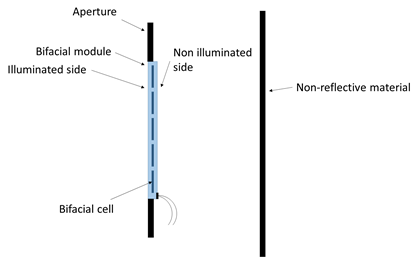Figure 1: Schematic of bifacial PV module and the required non-irradiated background and aperture.

To measure the irradiance on the non-exposed side, at least 5 points have to be chosen on the back side of the bifacial PV device, with symmetrical distribution. In the case of bifacial PV bare cells, background compensation can be performed by extrapolating the short-circuit current as a function of the background irradiance, since it may not be realistic to reach irradiance values below 3 Wm-2 for the background.

In order to determine the bifaciality coefficients of a PV device, the main I-V characteristics of the front and the rear sides must be measured at STC, using the requirements for the non-irradiated background described above. Using these measurements, bifacial parameters can be defined. The short-circuit current bifaciality coefficient φIsc is the ratio between the short-circuit current at STC generated exclusively by the rear side of the bifacial device and the one generated by the front side: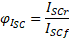(1)

Where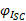is the short circuit current bifaciality coefficient (usually expressed as a percentage),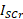is the short circuit current generated when the device is illuminated only on the rear side and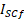is the short circuit current generated when the device is illuminated only on the front side. Unless it is known that the front and back spectral response of the device is identical, a spectral mismatch correction should be applied according to IEC 60904-7 . Bifaciality coefficients for open circuit voltage VOC and maximum power Pmax  can be calculated: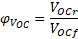(2)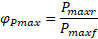(3)

Where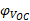is the open-circuit voltage bifaciality coefficient and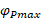is the maximum power bifaciality coefficient.  Apart from the calculation of the above parameters, the power generation gain due to the bifaciality of the device needs to be determined as a function of rear side irradiance.

# 3. BiFi measurements and calculations

## 3.1  Single-sided illumination systems: GE method

For single-sided illumination systems, Pmax of the device is measured at equivalent irradiance levels corresponding to 1000 Wm–2 front side plus the equivalent amount of front side irradiance corresponding to the rear side irradiance level GR(i). The total equivalent irradiance levels GE(i) are determined as functions of the bifaciality coefficient φ: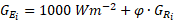(4)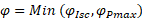(5)

It has to be noted that as the IEC 60904-1-2 describes, the φ value used to calculate the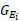values is the minimum between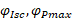.

In order to calculate the BiFi parameter, which expresses the bifacial power generation gain, Pmax of the device under test needs to be measured for at least two different equivalent irradiance levels, GE(i) (=1,2,3) (a third point is the Pmax(STC) already measured). BiFi is then defined as the linear fit’s slope of the Pmax (i) versus GR(i) data series, with the linear least squares fit forced to cross the Pmax axis at Pmax(STC). Since the resulting curve is a straight line, any non-linearities have to be considered in the uncertainty budget.

After calculating BiFi, there are two additional parameters that need to be reported: PmaxBiFi10 and PmaxBiFi20, for rear irradiance levels of GR1 = 100 Wm–2 and GR2 = 200 Wm–2 respectively. It should be highlighted that PmaxBiFi10 and PmaxBiFi20 must be obtained by linear interpolation of the data series Pmax(i) versus GR(i), based on the formulas: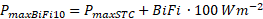(6)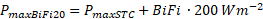(7)

## 3.2 Double-sided illumination systems

When a double-sided illumination system is used, the GR levels can be set directly by the double-sided system for acquiring the BiFi parameter. Along with the Pmax at STC measurement, Pmax of the bifacial PV device is measured at additional points, with 1000 Wm-2 on the front side plus rear side irradiance GR(i) (i=1,2,3,…), where at least two different values for GR(i) have to be used, with the recommended ones being GR(1)=100 Wm-2 and GR(2)=200 Wm-2. Similar to the single-sided illumination procedure, BiFi is then defined as the linear fit’s slope of the Pmax (i) versus GR(i) data series, with the linear least squares fit forced to cross the Pmax axis at Pmax(STC). Since the resulting curve is a straight line, any non-linearities have to be considered in the uncertainty budget.

PmaxBiFi10 and PmaxBiFi20, for rear irradiance levels of GR1 = 100 Wm–2 and GR2 = 200 Wm–2 respectively have to be reported as well, must be obtained by linear interpolation of the data series Pmax(i) versus GR(i), based on the formulas (6) and (7).

## 3.3 Example of Bifi measurements and calculations

It is assumed that a bifacial PV module is measured using a single-sided illumination system, applying the GE method. The measured values for STC power and bifaciality coefficients are  Pmax(STC)=400 W and φIsc=75 % and φPmax=76 %. Using these values, the equivalent GE(i) irradiances can be calculated for selected GR(i) assumed rear illumination. Since φIsc is the lowest value between φIsc and φPmax, it will be used for calculating the GE irradiance levels. Apart from STC conditions, three additional irradiance levels are selected for GR(100 Wm-2, 150 Wm-2, 200 Wm-2). For these assumed GR values, the equivalent irradiance GE is calculated according to equation (4). The results for the GE values are presented in Table 1. These GE values are set as the actual irradiance levels in order to acquire Pmax measurements of the module. It is assumed that these measurements yield the results presented in Table 1.

Table 1. GE values,  GR values and Pmax assumed measured values for the example.

 GR (Wm-2) GE (Wm-2) Pmax (W) 0 1000 400 100 1075 426.1 150 112.5 443.3 200 1150 454.1

Using these results, the BiFi parameter can be calculated by drawing the graph of GR versus Pmax. The graph is presented in Figure 2. As described earlier, linear fit is applied and the slope of the line is the bifacial power generation gain BiFi. The line is forced to intercept the point (0, Pmax(STC)). The results for BiFi, PmaxBiFi10 and PmaxBiFi20 from equations (6) and (7) are presented in Table 2.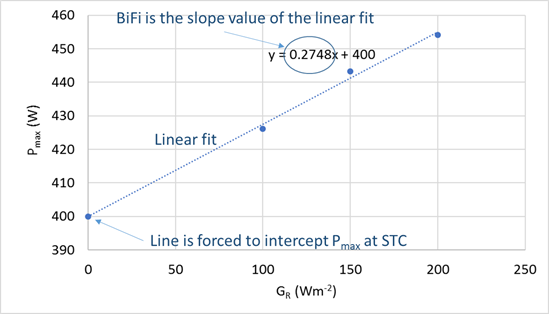Figure 2: Graph of Pmax as a function of GR, for calculation of the BiFi parameter, by the slope of the linear fit line for the measured points. The line is forced to intercept the point (0, Pmax(STC)).

Table 2. the values of BiFi, PmaxBiFi10 and PmaxBiFi20 calculated for the example

 BiFi (W/Wm-2) PmaxBiFi10 (W) PmaxBiFi20 (W) 0.275 427.5 455

Some specific steps of the above methodology have to be highlighted:

·  The GR data series is used for the BiFi parameter calculation and not the GE data series. This should potentially be made clearer in the IEC 60904-1-2:2019 document.

·  The PmaxBiFi10 and PmaxBiFi20 values are calculated by equations (6) and (7), even in the case that GR1=100 Wm-2 and GR2=200 Wm-2 are selected as measurement points. The calculated points may be marginally different from the measured points.

·  Uncertainties are ignored in the above example for simplicity, but in reality they need to be considered.

·  The above methodology would be identical for double-sided systems, with the difference that no GE calculations are required, since the front irradiance is constant at 1000 Wm-2.

References

 IEC 60904-1-2, “Photovoltaic devices - Part 1-2: Measurement of current-voltage characteristics of bifacial photovoltaic (PV) devices,” 2019.

 IEC 60904-9, “Photovoltaic devices – Part 9: Solar simulator performance requirements,” 2007.

 IEC 60904-7, “Photovoltaic devices – Part 7: Computation of the spectral mismatch correction for measurements of photovoltaic devices,” 2019.